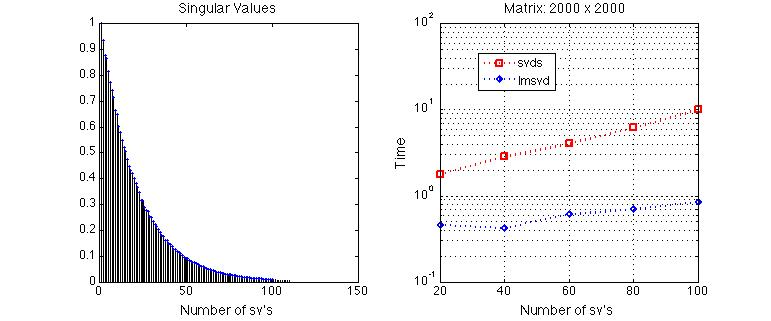# LMSVD

Limited Memory Block Krylov Subspace Optimization
for Computing Dominant Singular Value Decompositions

## Introduction

LMSVD is a MATLAB solver for computing dominant singular value decompositions of large matrices. The approach is based on a block Krylov subspace optimization technique which significantly accelerates the classic simultaneous iteration method.

Consider a real matrix $$A\in R^{m\times n}$$ ( $$m \le n$$ without loss of generality) and a given positive integer $$k \le \min(m,n)$$. The task is to find two orthogonal matrices $$U_k=[u_1,\ldots,u_k]\in R^{m\times k}$$ and $$V_k=[v_1,\ldots,v_k]\in R^{n\times k}$$, and a diagonal matrix $$\Sigma_k\in R^{k\times k}$$ whose diagonal entries are the $$k$$ largest singular values of $$A$$, say, $$\sigma_1 \geq\cdots \geq\sigma_{k} \ge 0$$, such that

$A_k := U_k \Sigma_k V_k^\top= \sum_{i=1}^k \sigma_i u_i v_i^\top = {\arg\min_{rank(W)\le k} \; \|A-W\|_F}$

The approximation $$A_k$$ is called the $$k$$-th dominant singular value decomposition of $$A$$.

## Publication

Xin Liu, Zaiwen Wen and Yin Zhang, Limited Memory Block Krylov Subspace Optimization for Computing Dominant Singular Value Decompositions, SIAM Journal on Scientific Computing, 35-3 (2013), A1641-A1668.

In the case that LMSVD is helpful in your published work, please make a reference to the above paper.

### Installation

• Unzip the distribution. This will create a directory called LMSVD. We'll refer to this directory as <lmsvd-root>.

• To verify the LMSVD installation, execute the following command within Matlab:

>> demo_lmsvd

### A Typical Demo Result(The figure in the left-hand side is the distribution of relevant singular values of a random test matrix in our demo, and the right-hand side is the runtime comparison between Matlab 'svds’ and 'lmsvd’ in calculating 20 to 100 dominant singular values of the test matrix.)

## Known Issues

The performance of LMSVD may be determined by the efficiency of the matrix-matrix multiplications AX.

• In Matlab 2013b, dense linear algebra operations have been generally well optimized by using BLAS and LAPACK tuned to the CPU processors in use. On the other hand, we have observed that some sparse linear algebra operations in Matlab 2013b seem to have not been as highly optimized. In particular, when doing multiplications between a sparse matrix and a dense vector or matrix (denoted by ‘‘SpMM’’) the performance of Matlab's own version of SPMM can differ significantly from that of the corresponding routine in Intel's Math Kernel Library (MKL), which is named ‘‘mkl_dcscmm’’.

• Comparison Results for Matrix Completion in the code SVT. LMSVD with SpMM-MKL are faster than with SpMM-Matlab.SpMM-MatlabSpMM-MKL

The detailed explanation is referred to section 5.2 in “X. Liu, Z. Wen, Y. Zhang, An Efficient Gauss-Newton Algorithm for Symmetric Low-Rank Product Matrix Approximations (pdf)